# How to write a greater than symbol(>) in LaTeX?

In this tutorial, we will discuss how to represent greater than symbols in a document with the help of latex.

Since greater than symbol is present on your keyboard. So, you don’t need to use any command separately for this.

Properties Value
Symbol Greater than
Package default
Argument No
Command >
Example >

You can represent this greater than symbol in a latex document by typing on the keyboard.

\documentclass{article}
\begin{document}
$$p > q$$
$$8 > 5$$
\end{document}

Output :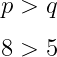## Greater than with an equal or not equal symbol

You have noticed that most of the time greater than symbol is used with equal and not equal symbols.

\documentclass{article}
\begin{document}
$$p \ge q$$
$$a \geq b$$
\end{document}

Output :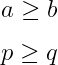Also, you need to install the amssymb package for the remaining symbols given below.

\documentclass{article}
\usepackage{amssymb}
\begin{document}
$$p \geqslant q$$
$$p \gneq$$
$$a \geqq b$$
$$a \gneqq b$$
\end{document}

Output :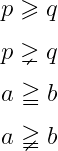## Greater greater than symbol in LaTeX

Mathematically, Greater greater than symbols are denoted by two and sometimes three greater than symbols.

\documentclass{article}
\usepackage{amssymb}
\begin{document}
$$p \gg q$$
$$p \ggg q$$
$$a \gggtr b$$
\end{document}

Output :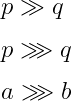You notice the latex program above, \ggg and \gggtr commands are used to represent the Greater greater symbol, which consists of three greater than symbols.

## Greater than with another symbols

Also, different types of symbols are used with greater than symbols. Such as less than, approximately, similar, etc.

If you define all the symbols differently, the tutorial will be much bigger. So, we will take the help of the matrix.

\documentclass{article}
\usepackage{amssymb}
\begin{document}
$$\begin{matrix} \eqslantgtr & \gtrsim & \gtrapprox \\ \gtreqqless & \gtreqless & \gtrless \end{matrix}$$
\end{document}

Output :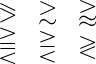You can add the not symbol with most of the symbols you have seen used above.

\documentclass{article}
\usepackage{amssymb}
\begin{document}
$$\begin{matrix} \ngtr & \ngeq & \ngeqslant \\[6pt] \ngeqq & \gnsim & \gnapprox \end{matrix}$$
\end{document}

Output :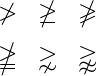And the use of these symbols above is much less but it is good for you to know.#### Md Jidan Mondal

LaTeX expert with over 10 years of experience in document preparation and typesetting. Specializes in creating professional documents, reports, and presentations using LaTeX.